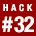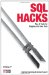# Hack 32. Cope with Rounding ErrorsReports sometimes include values that need to be rounded. You can do the rounding in your program or on the database server, but you need to be consistent.

Suppose you are required to produce a bill such as that shown in Table 5-11. You must add a 4 percent surcharge to every item. For each of the three items, the Surcharge column shows 4 percent of the price. In the Total row you have calculated the sum of the surcharges, not 4 percent of the total price.

Table 5-11. Bill with sum of surcharge

Item Price 4% Surcharge
Awl 100.10 4.00
Bowl 100.10 4.00
Cowl 800.80 32.03
Total 1,001.00 40.03

There is a discrepancy because a 4 percent charge on \$1,001.00 is \$40.04. But the bill shows the sum of the 4% Surcharge column as \$40.03.

The other way to calculate these values is to apply the 4 percent to every column including the Total, as shown in Table 5-12.

Table 5-12. Bill with percent calculation on total

Item Price 4% Surcharge
Awl 100.10 4.00
Bowl 100.10 4.00
Cowl 800.80 32.03
Total 1,001.00 40.04

You can have either the percentage calculation correct or the sum calculation correct. You can't have both be correct. When presenting data like this, the discrepancy in the sum is easier to spot by inspection than the discrepancy in the percentage. For this reason, it is common to sum the surcharges rather than calculate the surcharge on the sum. But you can do either.

Here is the calculation for each item:

```mysql> SELECT item
-> ,ROUND(net,2) AS Net
-> ,ROUND(net*0.04,2) AS Charge
-> FROM line;
+------+--------+--------+
| item | Net | Charge |
+------+--------+--------+
| Awl | 100.10 | 4.00 |
| Bowl | 100.10 | 4.00 |
| Cowl | 800.80 | 32.03 |
+------+--------+--------+```

Here are the two ways to calculate the total surcharge.

1. ROUND the SUM:
2. mysql> SELECT ROUND(SUM(net),2) AS Net -> ,ROUND(SUM(net*0.04),2) AS Charge -> FROM line; +---------+--------+ | Net | Charge | +---------+--------+ | 1001.00 | 40.04 | +---------+--------+
3. SUM the ROUND values:
4. mysql> SELECT SUM(ROUND(net,2)) AS Net -> ,SUM(ROUND(net*0.04,2)) AS Charge -> FROM line; +---------+--------+ | Net | Charge | +---------+--------+ | 1001.00 | 40.03 | +---------+--------+SQL Hacks
ISBN: 0596527993
EAN: 2147483647
Year: 2004
Pages: 147